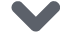# 我们能比卷积神经网络做得更好吗？

### 1. 层次图

def compute_iou_binary(seg1, seg2):    inters = float(np.count_nonzero(seg1 & seg2))    #区域可以预先计算    seg1_area = float(np.count_nonzero(seg1))    seg2_area = float(np.count_nonzero(seg2))    return inters / (seg1_area + seg2_area - inters)def hierarchical_graph(masks_multiscale, n_sp_actual, knn_graph=32):    n_sp_total = np.sum(n_sp_actual)    A = np.zeros((n_sp_total, n_sp_total))    for level1, masks1 in enumerate(masks_multiscale):        for level2, masks2 in enumerate(masks_multiscale[level1+1:]):            for i, mask1 in enumerate(masks1):                for j, mask2 in enumerate(masks2):                    A[np.sum(n_sp_actual[:level1], dtype=np.int) + i,                     np.sum(n_sp_actual[:level2+level1+1], dtype=np.int) + j] = compute_iou_binary(mask1, mask2)    sparsify_graph(A, knn_graph)    return A + A.Tn_sp_actual = []avg_values_multiscale, coord_multiscale, masks_multiscale = [], [], []# Scales [1000, 300, 150, 75, 21, 7] ]在论文中for i, (name, sp) in enumerate(zip(['children', 'parents', 'grandparents'], [1000, 300, 21])):    superpixels = slic(img, n_segments=sp)    n_sp_actual.append(len(np.unique(superpixels)))    avg_values_, coord_, masks_ = superpixel_features(img, superpixels)    avg_values_multiscale.append(avg_values_)    coord_multiscale.append(coord_)    masks_multiscale.append(masks_)A_spatial_multiscale = spatial_graph(np.concatenate(coord_multiscale), img.shape[:2], knn_graph=knn_graph)A_hier = hierarchical_graph(masks_multiscale, n_sp_actual, knn_graph=None)

### 2.易学的关系

pythonclass GraphLayerFusion(GraphLayerMultiscale):    def __init__(self,                 in_features,                 out_features,                 K,                 fusion='pc',                 n_hidden=64,                 bnorm=True,                 activation=nn.ReLU(True),                 n_relations=1):        super(GraphLayerFusion, self).__init__(in_features, out_features, K, bnorm, activation, n_relations)        self.fusion = fusion        if self.fusion == 'cp':            fc = [nn.Linear(in_features * K * n_relations, n_hidden),                  nn.ReLU(True),                  nn.Linear(n_hidden, out_features)]        else:            if self.fusion == 'pc':                fc = [nn.Linear(n_hidden * n_relations, out_features)]            elif self.fusion == 'sum':                fc = [nn.Linear(n_hidden, out_features)]            else:                raise NotImplementedError('cp, pc or sum is expected. Use GraphLayer for the baseline concatenation fusion')            self.proj = nn.ModuleList([nn.Sequential(nn.Linear(in_features * K, n_hidden), nn.Tanh())                                       for rel in range(n_relations)]) # projection layers followed by nonlinearity        if bnorm:            fc.append(BatchNorm1d_GNN(out_features))        if activation is not None:            fc.append(activation)        self.fc = nn.Sequential(*fc)    def relation_fusion(self, x, A):        B, N = x.shape[:2]        for rel in range(self.n_relations):            y = self.chebyshev_basis(A[:, :, :, rel], x, self.K).view(B, N, -1) # B,N,K,C            if self.fusion in ['pc', 'sum']:                y = self.proj[rel](y) # projection                if self.fusion == 'sum':                    y_out = y if rel == 0 else y_out + y                    continue            # for CP and PC            if rel == 0:                y_out = []            y_out.append(y)                y = self.fc(y_out if self.fusion == 'sum' else (torch.cat(y_out, 2))) # B,N,F        return y

### 结语点击下方 |  | 了解更多